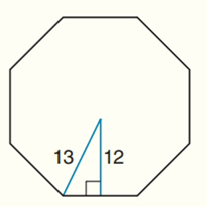Chapter 7.CT, Problem 14CTElementary Geometry For College St...

7th Edition
Alexander + 2 others
ISBN: 9781337614085

Solutions

Chapter
SectionElementary Geometry For College St...

7th Edition
Alexander + 2 others
ISBN: 9781337614085
Textbook Problem

For a regular octagon, the length of the apothem is approximately 12 cm and the length of the radius is approximately 13 cm. To the nearest centimeter, find the perimeter of the regular octagon.To determine

To find:

The perimeter of the regular octagon.

Explanation

Given:

The length of the apothem of the regular octagon = 12 cm.

The length of the radius = 13 cm

Calculation:

To find: the perimeter of the regular octagon.

The perimeter of the octagon is 8 × (length of one side of octagon).

Let us take the triangle, GSO.

We have to find the length of one side of octagon (GF).

Let the length of one side of octagon (GS) = x

GF = GS+SF

GF=x+xGF=2x

By using Pythagorean theorem:

If a and b are the lengths of the legs of a right triangle and c is the length of the hypotenuse, then the sum of the squares of the lengths of the legs is equal to the square of the length of the hypotenuse.

This relationship is represented by the formula a2=b2+c2.

Still sussing out bartleby?

Check out a sample textbook solution.

See a sample solution

The Solution to Your Study Problems

Bartleby provides explanations to thousands of textbook problems written by our experts, many with advanced degrees!

Get Started

Differentiate. y=ex1ex

Single Variable Calculus: Early Transcendentals, Volume I